### Adding a terrain Subtract node

This node subtracts one terrain from another terrain. At each vertex, the value of the output terrain is the difference of the values of both input terrains.

To add a node, right-click in the Graph Editor and select Create Node  > Terrain Composition > Subtract.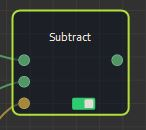This node has no prooerties.

### Linking to input terrains

Link the Subtract node to two input nodes.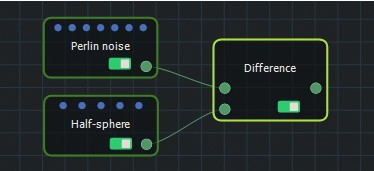If both input terrains are not the same size or the same resolution, the size and the resolution of the output terrain is determined by those of the first terrain, and the second terrain is enlarged or reduced so that its size matches the size of the output terrain exactly before being subtracted from the first terrain.

For example: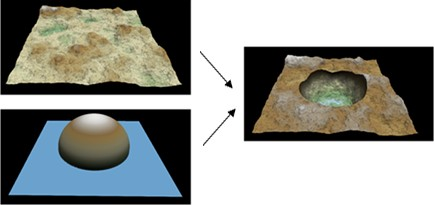### Linking to input terrains and a mask

Link the Difference using mask node to three input nodes.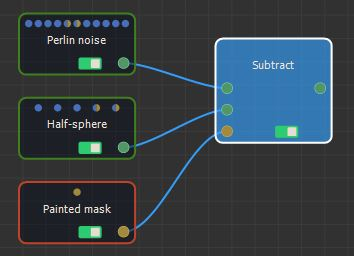At each vertex, if the value of the mask is equal to 1 (white color), the second terrain is subtracted from the first terrain. If the value of the mask is equal to 0 (black color), the height of the output terrain is equal to that of the first input terrain. The second input terrain is therefore ignored for this vertex. If the value of the mask is between 0 and 1, the height of the second input terrain is multiplied by the value of the mask before being added to the height of the first input terrain.

For example: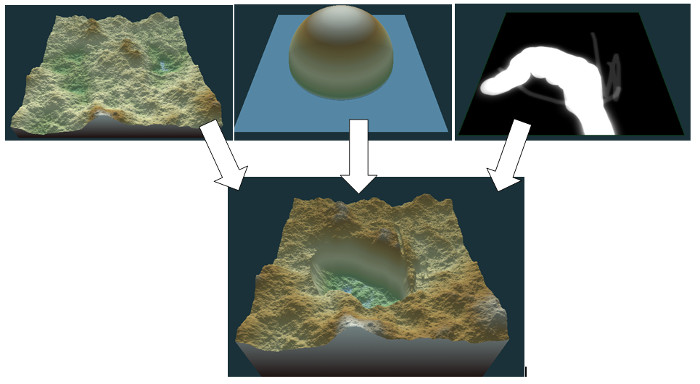Both input terrains and the input mask can have different sizes and resolutions. The size and resolution of the output terrain are determined by those of the first terrain and the second terrain and the mask are enlarged or reduced so that their size corresponds exactly to the size of the output terrain.

Copyright © 2021 · All Rights Reserved · Wysilab